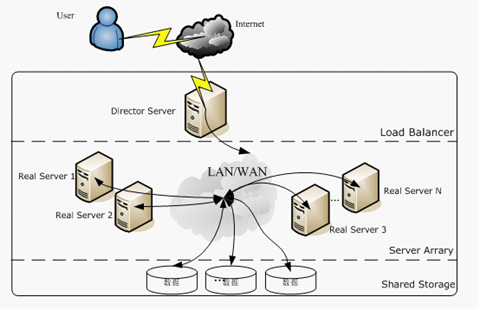# 机器学习之特征选择方法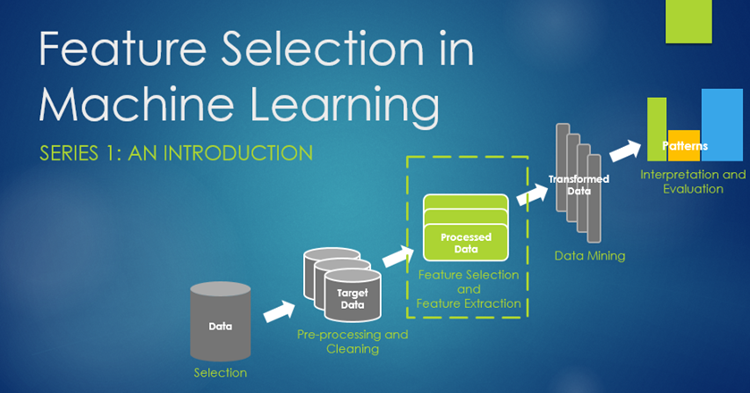• 收集更多数据
• 通过正则化引入对复杂度的惩罚
• 选择更少参数的简单模型
• 对数据降维（降维有两种方式：特征选择和特征抽取）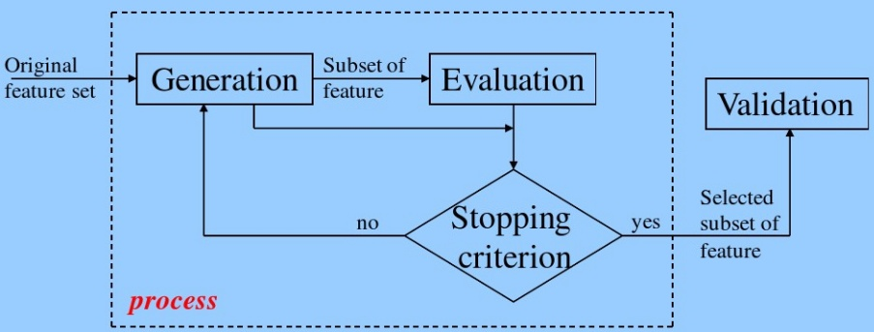1. 生成子集：搜索特征子集，为评价函数提供特征子集
2. 评价函数：评价特征子集的好坏
3. 停止准则：与评价函数相关，一般是阈值，评价函数达到一定标准后就可停止搜索
4. 验证过程：在验证数据集上验证选出来的特征子集的有效性

• 过滤类方法采用代理指标，而不根据特征子集的错误率计分。所选的指标算得快，但仍然能估算出特征集好不好用。常用指标包括互信息、逐点互信息、皮尔逊积矩相关系数、每种分类/特征的组合的帧间/帧内类距离或显著性测试评分。过滤类方法计算量一般比包装类小，但这类方法找到的特征子集不能为特定类型的预测模型调校。由于缺少调校，过滤类方法所选取的特征集会比包装类选取的特征集更为通用，往往会导致比包装类的预测性能更为低下。不过，由于特征集不包含对预测模型的假设，更有利于暴露特征之间的关系。许多过滤类方法提供特征排名，而非显式提供特征子集。要从特征列表的哪个点切掉特征，得靠交叉验证来决定。过滤类方法也常常用于包装方法的预处理步骤，以便在问题太复杂时依然可以用包装方法。
• 包装类方法使用预测模型给特征子集打分。每个新子集都被用来训练一个模型，然后用验证数据集来测试。通过计算验证数据集上的错误次数（即模型的错误率）给特征子集评分。由于包装类方法为每个特征子集训练一个新模型，所以计算量很大。不过，这类方法往往能为特定类型的模型找到性能最好的特征集。
• 嵌入类方法包括了所有构建模型过程中用到的特征选择技术。这类方法的典范是构建线性模型的LASSO方法。该方法给回归系数加入了L1惩罚，导致其中的许多参数趋于零。任何回归系数不为零的特征都会被LASSO算法“选中”。LASSO的改良算法有Bolasso和FeaLect。Bolasso改进了样本的初始过程。FeaLect根据回归系数组合分析给所有特征打分。 另外一个流行的做法是递归特征消除（Recursive Feature Elimination）算法，通常用于支持向量机，通过反复构建同一个模型移除低权重的特征。这些方法的计算复杂度往往在过滤类和包装类之间。

• Filter(过滤类)：按照发散性或相关性对各个特征进行评分，设定阈值或者待选择特征的个数进行筛选
• Wrapper(包装类)：根据目标函数（往往是预测效果评分），每次选择若干特征，或者排除若干特征
• Embedded(嵌入类)：先使用某些机器学习的模型进行训练，得到各个特征的权值系数，根据系数从大到小选择特征（类似于Filter，只不过系数是通过训练得来的）

## 过滤类方法

### Pearson相关系数

import numpy as np
from scipy.stats import pearsonr

np.random.seed(0)
size = 300
x = np.random.normal(0, 1, size)
print("Lower noise：", pearsonr(x, x + np.random.normal(0, 1, size)))
print("Higher noise：", pearsonr(x, x + np.random.normal(0, 10, size)))


• SelectKBest：留下topK高分的features
• SelectPercentile：留下百分比的top高分features
• 为每个feature都使用常用的单变量统计检验（univariate statistical tests）：
• SelectFpr（FPR: false positive rate，假阳，即负正本判为正），
• SelectFdr（FDR: false discovery rate，伪发现率），
• SelectFwe（FWER: family wise error，多重比较谬误）
• GenericUnivariateSelect：可以使用一个可配置的策略来进行单变量特征选择（univariate feature selection）。它允许你在超参数查找中选择最好的单变量选择策略

import numpy as np
from scipy.stats import pearsonr
from sklearn.feature_selection import SelectKBest

# 选择K个最好的特征，返回选择特征后的数据
# 第一个参数为计算评估特征是否好的函数，该函数输入特征矩阵和目标向量
# 输出二元组（评分，P值）的数组
# 数组第i项为第i个特征的评分和P值。在此定义为计算相关系数
# 参数k为选择的特征个数
def multivariate_pearsonr(X, y):
scores, p_values = [], []
for ret in map(lambda x: pearsonr(x, y), X.T):
print(ret)  # 输出每列的相关系数和p_value值
scores.append(abs(ret))
p_values.append(ret)
return (np.array(scores), np.array(p_values))

transformer = SelectKBest(score_func=multivariate_pearsonr, k=2)
X_new = transformer.fit_transform(iris.data, iris.target)
print(X_new)  # 输出具体特征列


Pearson相关系数的一个明显缺陷是，作为特征排序机制，他只对线性关系敏感。如果关系是非线性的，即便两个变量具有一一对应的关系，Pearson相关性也可能会接近 0。尽管Pearson相关系统存在这样的问题，同时存在如最大互信息系数、距离相关系数等方法，但当变量之间的关系接近线性相关的时候，Pearson相关系数仍然是不可替代的。

• Pearson相关系数计算速度快，这在处理大规模数据的时候很重要。
• Pearson相关系数的取值区间是[-1，1]，而MIC和距离相关系数都是[0，1]。这个特点使得Pearson相关系数能够表征更丰富的关系，符号表示关系的正负，绝对值能够表示强度。当然，Pearson相关性有效的前提是两个变量的变化关系是单调的。

### 卡方检验

from sklearn.datasets import load_iris
from sklearn.feature_selection import SelectKBest
from sklearn.feature_selection import chi2

X, y = iris.data, iris.target
# 选择K个最好的特征，返回选择特征后的数据
X_new = SelectKBest(chi2, k=2).fit_transform(X, y)
print(X_new)


### 距离相关系数（Distance Correlation）

$$\hat{d}\text{corr}(u, v)=\frac{\hat{d} \text{cov}(u, v)}{\sqrt{\hat{d} \text{cov}(u, u) \hat{d}\text{cov}(v, v)}}$$

$$\hat{d}\text{corr}^2(u, v)=\hat{S_1}+\hat{S_2}-2\hat{S_3}$$

$$\hat{S_1} = \frac{1}{n^2}\sum_{i=1}^{n}\sum_{j=1}^{n}\left \| u_i-u_j \right \|_{d_u}\left \| v_i-v_j \right \|_{d_v}$$

$$\hat{S_2} = \frac{1}{n^2}\sum_{i=1}^{n}\left \| u_i-u_j \right \|_{d_u}\frac{1}{n^2}\sum_{j=1}^{n}\left \| v_i-v_j \right \|_{d_v}$$

$$\hat{S_3} = \frac{1}{n^3}\sum_{i=1}^{n}\sum_{j=1}^{n}\sum_{l=1}^{n}\left \| u_i-u_l \right \|_{d_u}\left \| v_i-v_l \right \|_{d_v}$$

from scipy.spatial.distance import pdist, squareform
import numpy as np
import copy

def distcorr(Xval, Yval, pval=True, nruns=500):
""" Compute the distance correlation function, returning the p-value."""
X = np.atleast_1d(Xval)
Y = np.atleast_1d(Yval)
if np.prod(X.shape) == len(X):
X = X[:, None]
if np.prod(Y.shape) == len(Y):
Y = Y[:, None]
X = np.atleast_2d(X)
Y = np.atleast_2d(Y)
n = X.shape
if Y.shape != X.shape:
raise ValueError('Number of samples must match')
a = squareform(pdist(X))
b = squareform(pdist(Y))
A = a - a.mean(axis=0)[None, :] - a.mean(axis=1)[:, None] + a.mean()
B = b - b.mean(axis=0)[None, :] - b.mean(axis=1)[:, None] + b.mean()

dcov2_xy = (A * B).sum() / float(n * n)
dcov2_xx = (A * A).sum() / float(n * n)
dcov2_yy = (B * B).sum() / float(n * n)
dcor = np.sqrt(dcov2_xy) / np.sqrt(np.sqrt(dcov2_xx) * np.sqrt(dcov2_yy))

if pval:
greater = 0
for i in range(nruns):
Y_r = copy.copy(Yval)
np.random.shuffle(Y_r)
if distcorr(Xval, Y_r, pval=False) > dcor:
greater += 1
return (dcor, greater / float(nruns))
else:
return dcor


from sklearn.datasets import load_iris
import pandas as pd
import numpy as np
import dcor

iris_df = pd.DataFrame(data=np.c_[iris['data'], iris['target']],
columns=iris['feature_names'] + ['target'])

print("dcor distance correlation = {:.3f}".format(
dcor.distance_correlation(iris_df['sepal length (cm)'], iris_df['petal length (cm)'])))


### 方差选择法（VarianceThreshold）

$$Var[X] = p(1 – p)$$

VarianceThreshold是特征选择的简单基线方法。它删除方差不符合某个阈值的所有特征。默认情况下，它会删除所有零差异特征，即所有样本中具有相同值的特征。代码如下：

from sklearn.feature_selection import VarianceThreshold

X = [[0, 0, 1], [0, 1, 0], [1, 0, 0], [0, 1, 1], [0, 1, 0], [0, 1, 1]]
# 方差选择法，返回值为特征选择后的数据
# 参数threshold为方差的阈值
sel = VarianceThreshold(threshold=(.8 * (1 - .8)))
print(sel.fit_transform(X)


from sklearn.feature_selection import VarianceThreshold

X_new = VarianceThreshold(threshold=2).fit_transform(iris.data)
print(X_new)


## 包装类方法

• 前向选择法：这是一种基于循环的方法，开始时我们训练一个不包含任何特征的模型，而后的每一次循环我们都持续放入能最大限度提升模型的变量，直到任何变量都不能提升模型表现。
• 后向剔除法：该方法先用所有特征建模，再逐步剔除最不显著的特征来提升模型表现。同样重复该方法直至模型表现收敛。
• 迭代剔除法：这是一种搜索最优特征子集的贪心优化算法。它会反复地训练模型并剔除每次循环的最优或最劣特征。下一次循环，则使用剩余的特征建模直到所有特征都被剔除。之后，按照剔除的顺序给所有特征排序作为特征重要性的度量。

### 递归特征消除法(Recursive feature elimination)

Sklearn的feature_selection库下RFE类可以用来选择特征，具体代码如下：

from sklearn.feature_selection import RFE
from sklearn.linear_model import LogisticRegression

# 递归特征消除法，返回特征选择后的数据
# 参数estimator为基模型
# 参数n_features_to_select为选择的特征个数
X_new = RFE(estimator=LogisticRegression(), n_features_to_select=2).fit_transform(iris.data, iris.target)
print(X_new)


## 嵌入类方法

• 过滤法测量特征和被解释变量的相关性，包装法则是基于模型测量特征的有效性。
• 过滤法由于不依赖于模型，速度更快。
• 过滤法基于统计检验选择特征，包装法基于交叉验证。
• 过滤法时常失效，但包装法常常被发现很有用。
• 使用包装法筛选的特征更容易导致模型过拟合。

### 基于惩罚项的特征选择

SelectFromModel是一个元转换器（meta-transformer），可以用在任何在fitting后具有coef_或feature_importances_属性的estimator。如果相应的coef_ 或 feature_importances_值在提供的参数threshold之下，那么这些不重要的features将被移除。除了指定一个数值型的threshold，还内置了些string参数作为阀值的探索法（heuristics）。这些heuristics方法有：“mean”, “median”以及浮点乘法（比如：“0.1*mean”）

from sklearn.svm import LinearSVC
from sklearn.feature_selection import SelectFromModel

X, y = iris.data, iris.target

lsvc = LinearSVC(C=0.01, penalty="l1", dual=False).fit(X, y)
model = SelectFromModel(lsvc, prefit=True)
X_new = model.transform(X)
print(X_new)

from sklearn.linear_model import LogisticRegression
from sklearn.feature_selection import SelectFromModel

# 带L1惩罚项的逻辑回归作为基模型的特征选择
X_new = SelectFromModel(LogisticRegression(penalty="l1", C=0.1)).fit_transform(iris.data, iris.target)
print(X_new)


from sklearn.linear_model import LogisticRegression
from sklearn.feature_selection import SelectFromModel

class LR(LogisticRegression):
def __init__(self, threshold=0.01, dual=False, tol=1e-4, C=1.0,
fit_intercept=True, intercept_scaling=1, class_weight=None,
random_state=None, solver='liblinear', max_iter=100,
multi_class='ovr', verbose=0, warm_start=False, n_jobs=1):

# 权值相近的阈值
self.threshold = threshold
LogisticRegression.__init__(self, penalty='l1', dual=dual, tol=tol, C=C,
fit_intercept=fit_intercept, intercept_scaling=intercept_scaling,
class_weight=class_weight,
random_state=random_state, solver=solver, max_iter=max_iter,
multi_class=multi_class, verbose=verbose, warm_start=warm_start, n_jobs=n_jobs)
# 使用同样的参数创建L2逻辑回归
self.l2 = LogisticRegression(penalty='l2', dual=dual, tol=tol, C=C, fit_intercept=fit_intercept,
intercept_scaling=intercept_scaling, class_weight=class_weight,
random_state=random_state, solver=solver, max_iter=max_iter,
multi_class=multi_class, verbose=verbose, warm_start=warm_start, n_jobs=n_jobs)

def fit(self, X, y, sample_weight=None):
# 训练L1逻辑回归
super(LR, self).fit(X, y, sample_weight=sample_weight)
self.coef_old_ = self.coef_.copy()
# 训练L2逻辑回归
self.l2.fit(X, y, sample_weight=sample_weight)

cntOfRow, cntOfCol = self.coef_.shape
# 权值系数矩阵的行数对应目标值的种类数目
for i in range(cntOfRow):
for j in range(cntOfCol):
coef = self.coef_[i][j]
# L1逻辑回归的权值系数不为0
if coef != 0:
idx = [j]
# 对应在L2逻辑回归中的权值系数
coef1 = self.l2.coef_[i][j]
for k in range(cntOfCol):
coef2 = self.l2.coef_[i][k]
# 在L2逻辑回归中，权值系数之差小于设定的阈值，且在L1中对应的权值为0
if abs(coef1 - coef2) < self.threshold and j != k and self.coef_[i][k] == 0:
idx.append(k)
# 计算这一类特征的权值系数均值
mean = coef / len(idx)
self.coef_[i][idx] = mean
return self

# 带L1和L2惩罚项的逻辑回归作为基模型的特征选择
# 参数threshold为权值系数之差的阈值
X_new = SelectFromModel(LR(threshold=0.5, C=0.1)).fit_transform(iris.data, iris.target)
print(X_new)


### 随机稀疏模型(Randomized sparse models)

$$\hat{w}_{I}=\arg \min _{w} \frac{1}{2 n_{I}} \sum_{i \in I}(y_{i}-x_{i}^{T} w)^{2}+\alpha \sum_{j=1}^{p} \frac{|w_{j}|}{s_{j}}$$

### 基于树的特征选择(Tree-based feature selection)

from sklearn.ensemble import RandomForestRegressor

X = iris["data"]
Y = iris["target"]
names = iris["feature_names"]

rf = RandomForestRegressor()
rf.fit(X, Y)
print("Features sorted by their score:")
print(sorted(zip(map(lambda x: "%.4f" % x, rf.feature_importances_), names), reverse=True))


• 这种方法存在偏向 ，对具有更多类别的变量会更有利；
• 对于存在关联的多个特征，其中任意一个都可以作为指示器（优秀的特征），并且一旦某个特征被选择之后，其他特征的重要度就会急剧下降(因为不纯度已经被选中的那个特征降下来了，其他的特征就很难再降低那么多不纯度了，这样一来，只有先被选中的那个特征重要度很高，其他的关联特征重要度往往较低)。在理解数据时，这就会造成误解，导致错误的认为先被选中的特征是很重要的，而其余的特征是不重要的，但实际上这些特征对响应变量的作用确实非常接近的（这跟Lasso是很像的）。特征随机选择方法稍微缓解了这个问题，但总的来说并没有完全解决。

from sklearn.model_selection import ShuffleSplit
from sklearn.metrics import r2_score
from collections import defaultdict
from sklearn.ensemble import RandomForestRegressor
import numpy as np

X = iris["data"]
Y = iris["target"]
names = iris["feature_names"]

rf = RandomForestRegressor()
scores = defaultdict(list)
# crossvalidate the scores on a number of different random splits of the data
for train_idx, test_idx in ShuffleSplit(len(X), 100, .3).split(X):
X_train, X_test = X[train_idx], X[test_idx]
Y_train, Y_test = Y[train_idx], Y[test_idx]
r = rf.fit(X_train, Y_train)
acc = r2_score(Y_test, rf.predict(X_test))
for i in range(X.shape):
X_t = X_test.copy()
np.random.shuffle(X_t[:, i])
shuff_acc = r2_score(Y_test, rf.predict(X_t))
scores[names[i]].append((acc - shuff_acc) / acc)
print("Features sorted by their score:")
print(sorted([(float('%.4f' % np.mean(score)), feat) for
feat, score in scores.items()], reverse=True))


from sklearn.ensemble import ExtraTreesClassifier
from sklearn.feature_selection import SelectFromModel

X, y = iris.data, iris.target

clf = ExtraTreesClassifier()
clf = clf.fit(X, y)
print(clf.feature_importances_)
model = SelectFromModel(clf, prefit=True)
X_new = model.transform(X)
print(X_new)


## 降维

### 主成分分析（PCA）

from sklearn.decomposition import PCA

# 主成分分析法，返回降维后的数据
# 参数n_components为主成分数目
X_new = PCA(n_components=2).fit_transform(iris.data)
print(X_new)


### 线性判别分析法（LDA）

from sklearn.discriminant_analysis import LinearDiscriminantAnalysis

# 线性判别分析法，返回降维后的数据
# 参数n_components为降维后的维数
X_new = LinearDiscriminantAnalysis(n_components=2).fit_transform(iris.data, iris.target)
print(X_new)


## 总结

• 对于理解数据、数据的结构、特点来说，单变量特征选择是个非常好的选择。尽管可以用它对特征进行排序来优化模型，但由于它不能发现冗余（例如假如一个特征子集，其中的特征之间具有很强的关联，那么从中选择最优的特征时就很难考虑到冗余的问题）。
• 正则化的线性模型对于特征理解和特征选择来说是非常强大的工具。L1正则化能够生成稀疏的模型，对于选择特征子集来说非常有用；相比起L1正则化，L2正则化的表现更加稳定，由于有用的特征往往对应系数非零，因此L2正则化对于数据的理解来说很合适。由于响应变量和特征之间往往是非线性关系，可以采用basis expansion(基展开)的方式将特征转换到一个更加合适的空间当中，在此基础上再考虑运用简单的线性模型。
• 随机森林是一种非常流行的特征选择方法，它易于使用，一般不需要feature engineering、调参等繁琐的步骤，并且很多工具包都提供了平均不纯度下降方法。它的两个主要问题，1是重要的特征有可能得分很低（关联特征问题），2是这种方法对特征变量类别多的特征越有利（偏向问题）。尽管如此，这种方法仍然非常值得在你的应用中试一试。
• 特征选择在很多机器学习和数据挖掘场景中都是非常有用的。在使用的时候要弄清楚自己的目标是什么，然后找到哪种方法适用于自己的任务；当选择最优特征以提升模型性能的时候，可以采用交叉验证的方法来验证某种方法是否比其他方法要好；当用特征选择的方法来理解数据的时候要留心，特征选择模型的稳定性非常重要，稳定性差的模型很容易就会导致错误的结论；数据进行二次采样然后在子集上运行特征选择算法能够有所帮助，如果在各个子集上的结果是一致的，那就可以说在这个数据集上得出来的结论是可信的，可以用这种特征选择模型的结果来理解数据。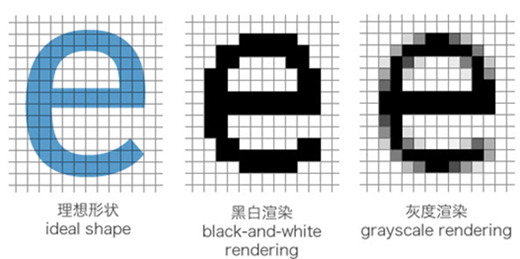##### 网站与APP开发中的字体设置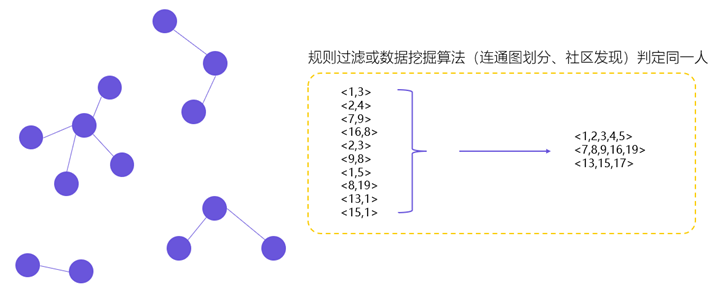##### 用户体系搭建之ID-Mapping